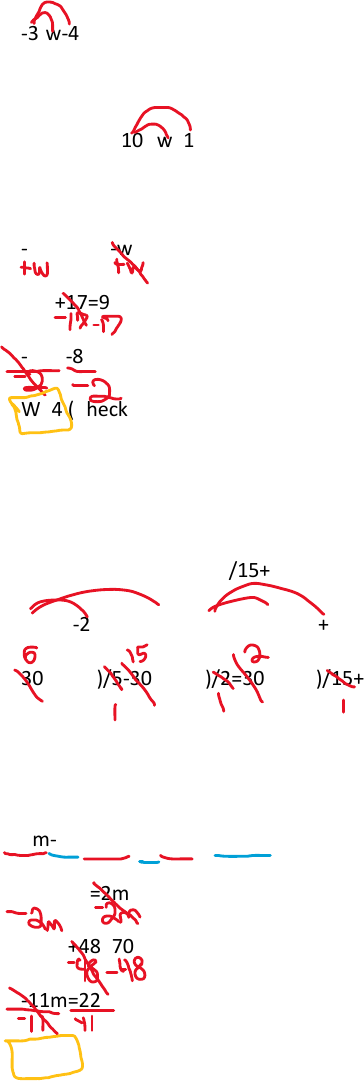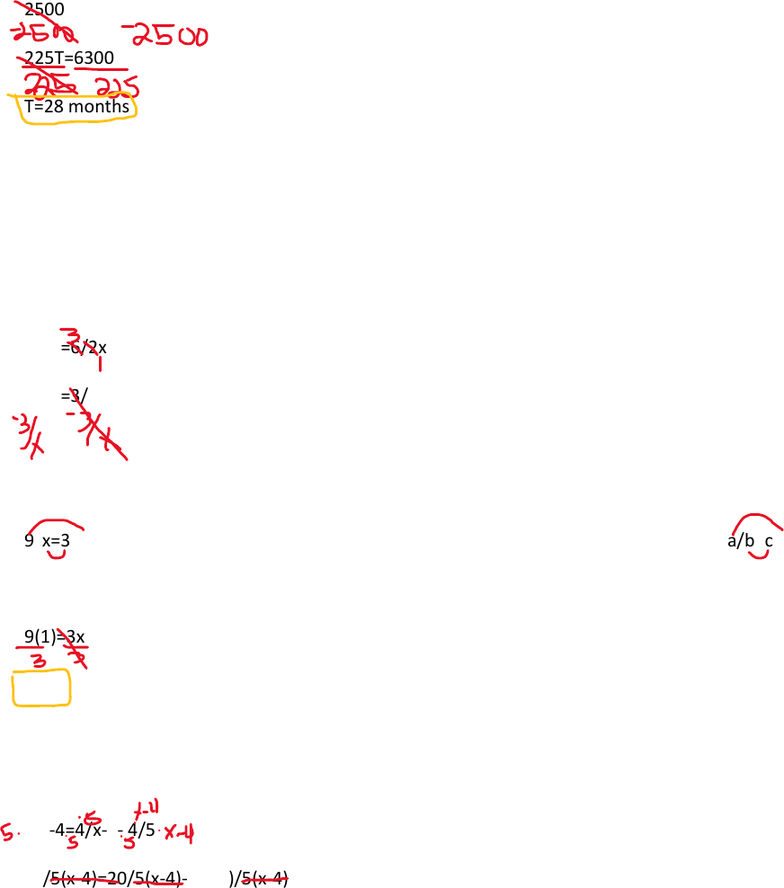Class Notes (1,100,000)
US (490,000)
WSU (30)
MAT (8)
Lecture 1

# MATH 111 Lecture Notes - Lecture 1: Memory Stick, Cross Product, American Broadcasting Company

This preview shows pages 1-2. to view the full 7 pages of the document.1.) Linear Equations- Solve 1.1, 8/20/2018
-3(w-4)+5=10-(w+1) w=unknown (distribute)
If it has an equal sign we know it is an equation and we solve equations.
-3w+12+5=10-(w+1) (distribute)
-3w+12+5=10-w-1 (simplify)
-3w+17=9-w (move the variable to the same side)
-2w+17=9 (move all the numbers to the same side)
-2w=-8
W=4 (Check)
Ex.) Solve-
(m-2)/5-(m-4)/2=(m+5)/15+2 (linear because the variable isn’t in the denominator)
30 (m-2/5 m-4/2)= 30 (m+5/15 +2)
30×(m-2)/5-30×(m-4)/2=30 (m+5)/15+30x2
6(m-2)-15(m+4)=2m+5+6(2)
Then distribute, combine like terms, and simplify
6m-12-15m+60=2m+10+60 Always do the opposite of what we are doing to simplify things
-9m+48=2m+70
-11m+48=70
-11m=22
M=-2 (Check)
Ex. A couple needs to decide whether to buy a used car for \$8,000 or lease a new car for an
initial down payment of of \$2,500 followed by 36 monthly payments of \$225.

Only pages 1-2 are available for preview. Some parts have been intentionally blurred.A.) Write a model for the cost C in dollars to lease the car for T months.
C= 2,500+225(T)
B.) After how many months will the cost to lease the new car be equal to the cost to buy a used
car?
+=# of months
2500+225T= 8800
225T=6300
T=28 months
2. Solving Rational Equations *rational bc X is in the denominator
Ex. Solve- *checking is mandatory here
12/x=6/2x+3
12/x=6/2x+3
12/x=3/x+3
12/x-3/x=3
9/x=3/1 *cross multiply (cross product a/b=c/d)
9(1)=3x
X=3 (check)
Ex. Solve and Check
x/(x-4)=4/(x-4)-4⁄5 (factored?) LCD= 5(x-4)
x/x-4=4/x-4- 4/5
5x/5(x-4)=20/5(x-4)-4(x-4)/5(x-4) LCD becomes the new denominator